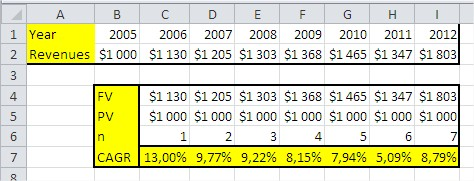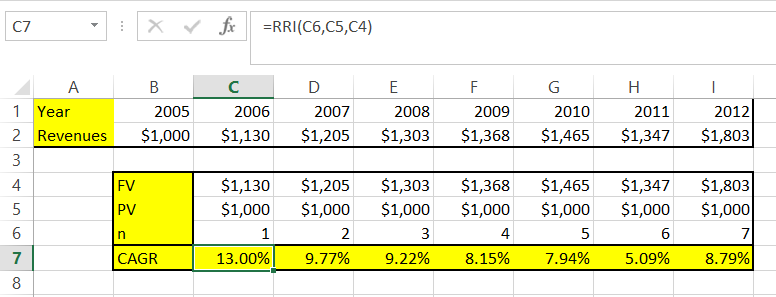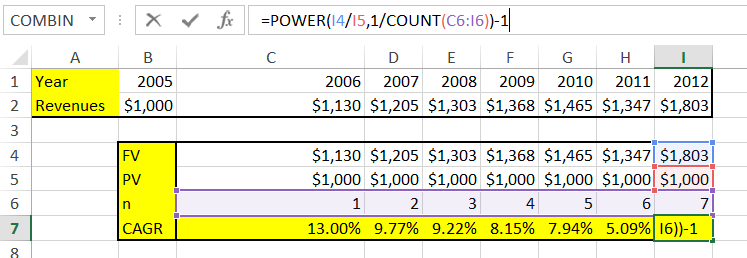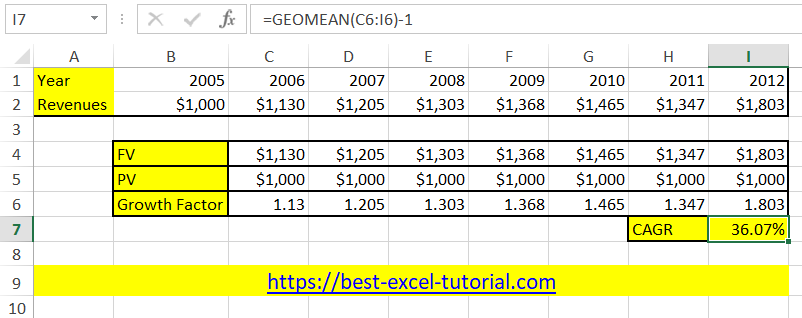#### How to Calculate Cagr in Excel

In this lesson, you will learn how to calculate CAGR in Excel.

## What is CAGR?

CAGR (Compound Annual Growth Rate) is the year-over-year average growth rate over a period of time. By calculating CAGR, you can check how much you earn annually with your investments.

There are several ways to calculate CAGR in Excel.

## Methods of CAGR calculations

### Method 1. Dedicated formula

To calculate CAGR, you can use that formula:

= ((FV/PV)^(1/n)) – 1

• FV stands for Future Value
• PV stands for Present Value
• n stands for number of investment yearsThe picture shows an example of the formula. I think everything is clear.

### Method 2. RRI function

The other way is to use the RRI Excel function.

RRI function takes 3 arguments:

• nper (the same as n in the previous example)
• PV
• FV

In such a situation, the function in the given example will be: =RRI(C6,C5,C4)The results are the same, so it proves that both of the methods are working the same way.

### Method 3. Power function

Calculating CAGR is possible with the Power function

The other way to calculate a Compound Annual Growth Rate is possible with a Power Excel function.

The formula would be like this: =POWER(I4/I5,1/COUNT(C6:I6))-1 which is in fact =POWER(FV/PV,1/NPER)-1Power function usage might be surprising to you, but the explanation is simple. To calculate a CAGR, you just need to calculate the rate of future value divided by present value. At this rate, you should power by the number of periods, which is 7 in our example. Of course, the result needs to be decreased by 1 to get the percentage value.

### Method 4. GEOMEAN function

Another example to calculate cagr is to use the cagr function.

The first step is to calculate the growth factor. It is simply the ratio of future value to present value. Growth factor is greater than zero when the future value is greater than the present value, and less than zero when the present value is greater.

Then, using the geomean function, we calculate the geometric mean of the growth factor. The CAGR is the geometric mean minus one.The full geomean formula is: =GEOMEAN(C6:I6)-1

### Method 5. IRR function

The IRR function calculates the internal rate of return, which can be interpreted as the CAGR of the investment.

`=IRR(cash_flows)`

### Method 6. XIRR function

The XIRR function is used for investments with irregular cash flows and considers the timing of each cash flow.

`=XIRR(cash_flows, dates)`

### Method 7. RATE function

The RATE function calculates the interest rate for investments with regular cash flows.

`=RATE(nper, pmt, PV, FV)`